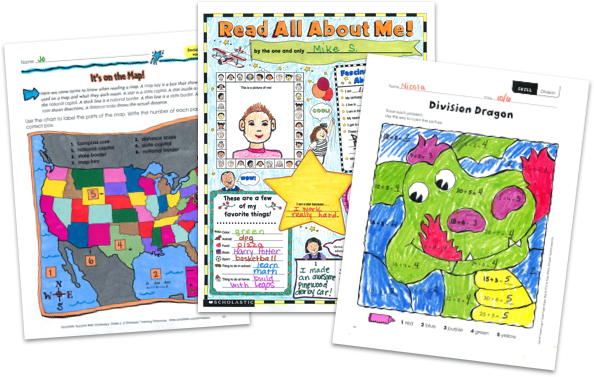MY FILE CABINET# 8th Grade Math Worksheets From Scholastic TeachablesScholastic Teachables (formerly Scholastic Printables) has more than 100 eighth grade math worksheets that span more than 20 different math skills. Sign up today and get instant online access to 8th grade math worksheets, plus lesson plans, practice pages, and more to cover all skills. You'll also get access to Scholastic's 30,000+ award-winning printables and leveled learning collections in reading and math for all grade levels. Learn why thousands of teachers have chosen Scholastic to be their teaching resource.

Scholastic Teachables (formerly Scholastic Printables) has more than 100 eighth grade math worksheets that span more than 20 different math skills. Sign up today and get instant online access to 8th grade math worksheets, plus lesson plans, practice pages, and more to cover all skills. You'll also get access to Scholastic's 30,000+ award-winning printables and leveled learning collections in reading and math for all grade levels. Learn why thousands of teachers have chosen Scholastic to be their teaching resource.

## Scholastic's 8th Grade Math Worksheets Cover:

• Algebra
• Averages
• Charts and Graphs
• Data Analysis
• Decimals
• Fractions
• Functions
• Geometry
• Inequalities
• Life-Skills Math
• Logic and Problem Solving
• Measures of Central Tendency
• Money
• Multiplication and Division
• Patterns
• Percents
• Permutations
• Probability and Statistics
• Properties
• Ratio, Proportion, and Scale
• Real-World Math
• Simulations
• Unit Rates
• Word Problems

## Sample 8th Grade Math Worksheets

More than 100 printable 8th grade math worksheets from Scholastic Teachables span more than 20 math topics and skills. Sign up and you'll also get access to Scholastic's more than 30,000 award-winning printables and leveled learning collections in reading and math to reach every learner. New printables and 8th grade math worksheets are published each month to keep your courses fresh, exciting and up-to-date. You can access all of our resources from any device, whether at school or at home. Spend more time focusing on your courses and less time on preparing materials—simply find it, print it, teach it!

Here's a sample of 8th grade math worksheets for you to try in your class—FREE with a 30-day trial or subscription.

## Scholastic's 3rd Grade Math Worksheets Cover:

• Algebra
• Averages
• Charts and Graphs
• Data Analysis
• Decimals
• Fractions
• Functions
• Geometry
• Inequalities
• Life-Skills Math
• Logic and Problem Solving
• Measures of Central Tendency
• Money
• Multiplication and Division
• Patterns
• Percents
• Permutations
• Probability and Statistics
• Properties
• Ratio, Proportion, and Scale
• Real-World Math
• Simulations
• Unit Rates
• Word Problems
• Algebra
• Averages
• Charts and Graphs
• Data Analysis
• Decimals
• Fractions
• Functions
• Geometry
• Inequalities
• Life-Skills Math
• Logic and Problem Solving
• Measures of Central Tendency
• Money
• Multiplication and Division
• Patterns
• Percents
• Permutations
• Probability and Statistics
• Properties
• Ratio, Proportion, and Scale
• Real-World Math
• Simulations
• Unit Rates
• Word Problems

## Sample 8th Grade Math Worksheets

More than 100 printable 8th grade math worksheets from Scholastic Teachables span more than 20 math topics and skills. Sign up and you will also get access to Scholastic's more than 30,000 award-winning printables and leveled learning collections in reading and math to reach every learner. New printables and 8th grade math worksheets are published each month to keep your courses fresh, exciting and up-to-date. You can access all of our resources from any device, whether at school or at home. Spend more time focusing on your courses and less time on preparing materials—simply find it, print it, teach it!

Here's a sample of 8th grade math worksheets for you to try in your class—FREE with a 30-day trial or subscription.## Additional Alphabet Worksheets (Subscription Required)

Try FREE for 30 days!

If you are not 100% satisfied cancel during the FREE trial and owe nothing. If you are not 100% satisfied cancel during the FREE trial and owe nothing.